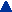Printing errors found in the manuscript are corrected to this WWW-page.
They are indicated with this green color.
In the original publication the figures are in black and white.

IEEE TRANSACTIONS ON BIOMEDICAL ENGINEERING, VOL. 44, NO. 3. MARCH 1997, pp. 196-208

# and MEG Measurements

Jaakko Malmivuo,* Senior Member IEEE, Veikko Suihko and Hannu Eskola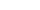Abstract - It is generally believed that because the skull has low conductivity to electric current but is transparent to magnetic fields, the measurement sensitivity of the magnetoencephalography (MEG) in the brain region should be more concentrated than that of the electroencephalography (EEG). It is also believed that the information recorded by these techniques is very different. If this were indeed the case, it might be possible to justify the cost of MEG instrumentation which is at least 25 times higher than that of EEG instrumentation. The localization of measurement sensitivity using these techniques was evaluated quantitatively in an inhomogeneous spherical head model using a new concept called half-sensitivity volume (HSV). It is shown that the planar gradiometer has a far smaller HSV than the axial gradiometer. However, using the EEG it is possible to achieve even smaller HSV's than with whole-head planar gradiometer MEG devices. The micro-superconducting quantum interference device (SQUID) MEG device does have HSV's comparable to those of the EEG. The sensitivity distribution of planar gradiometers, however, closely resembles that of dipolar EEG leads and therefore, the MEG and EEG record the electric activity of the brain in a very similar way.

Index Terms - Bioelectromagnetism, electroencephalography, magnetoencephalography.

Manuscript received September 28, 1995; revised October 17, 1996. This work was supported by the Academy of Finland and the Ragnar Granit Foundation. Asterix indicates corresponding author.
*J. Malmivuo is with the Ragnar Granit Institute, Tampere University of Technology, FIN-33101 Tampere, Finland (e-mail: jaakko.malmivuo@tut.fi).
V. Suihko and H. Eskola are with the Ragnar Granit Institute, Tampere University of Technology, FIN-33101 Tampere, Finland.
I. INTRODUCTIONWHEN electrically active tissue produces a bioelectric field, it simultaneously produces a biomagnetic field. Thus the origin of both the bioelectric and the biomagnetic signals is the bioelectric activity of the tissue.

Magnetic detection of the bioelectric activity introduces both technical and bioelectromagnetic differences compared to the electric method. One technical advantage of the magnetic method is that biomagnetic signals may be detected without attaching electrodes to the skin. Furthermore, superconducting SQUID detectors are capable of detecting dc currents. On the other hand, biomagnetic technology needs, especially in brain studies, very expensive instrumentation and a magnetically shielded room. Their cost is at least 25 times that of electroencephalography (EEG) instrumentation .
Bioelectromagnetic differences include differences in the information contents of the electric and magnetic signals and in the abilities of these methods to concentrate their measurement sensitivity or to localize electric sources.
The relative merits of the EEG and magnetoencephalography (MEG) have been a subject of very controversial discussion including articles on scientific experiments , , , scientific discussions , and editorial articles . With this paper we participate in this discussion. The results of this paper are calculated on the basis of the theory we have published previously  - , .
II. BIOELECTROMAGNETIC BACKGROUND

A. The concept of Lead Field

The bioelectromagnetic differences between EEG and MEG may be explained by the different sensitivity distributions of electric and magnetic measurement methods . We have used the lead field approach to calculate the sensitivity distributions of the EEG and MEG leads. The lead field is an electric current field in the volume conductor generated by feeding a unit current to the lead. (Because the volume conductor is not superconducting, in magnetic leads an alternating current having a unit time derivative is used.) According to the reciprocity theorem of Helmholtz , the current field produced in this manner in the volume conductor is identical to the distribution of the sensitivity of the lead.

B. Differences in the Information Contents of the EEG and the MEG

The initially optimistic view of the new information content of magnetic recordings was based on consideration of Helmholtz's theorem which states: "A general vector field, which vanishes at infinity, can be represented as the sum of two independent vector fields; one that is irrotational (zero curl) and another which is solenoidal (zero divergence)." These vector fields are often referred to as the "flow source" and the "vortex source", respectively.
In an idealized case where the head is modelled with concentric conducting spheres it can be shown that the electric field generated by the current sources of the brain arises from a flow source and the associated magnetic field from a vortex source. In the beginning of biomagnetic research it was believed that because of the Helmholtz Theorem, these two fields are independent and that as much new information can be obtained from magnetic recordings as is already present in electric recordings. However, experimental studies have demonstrated that these signals look very much the same and are not fully independent. This apparent contradiction can be resolved in the following way .
The sensitivity of a lead system which detects the dipolar term of the flow source consists of three orthogonal components, each of which is linear and homogeneous. The orthogonality means that none of them can be obtained as a linear combination of the other two. Thus, the three sensitivity distributions are fully independent. However, the electric signals each lead records cannot be completely independent because each represents a different aspect of the same volume source.
Similarly, the sensitivity distribution of a lead system for the detection of the dipolar term of the vortex source also has three orthogonal components. Each component can be represented by a set of concentric circles such that the lead sensitivity is always tangential to the symmetry axis. The magnitude of the sensitivity is proportional to the radial distance from the symmetry axis. Again, because the sensitivity distributions of these three components are orthogonal, none of them can be constructed as a linear combination of the other two. Thus, all three magnetic sensitivity distributions are also fully independent. However, as before, the three signals detected by the magnetic leads are not fully independent because each represents a different aspect of the same volume source.
It is now possible to resolve the paradox involving Helmholtz's Theorem. What the Helmholtz Theorem expresses is not the independence of electric and magnetic signals, but the independence of the sensitivity distributions of the recordings of the flow and vortex sources, i.e., the electric and magnetic lead fields. It indicates that the three electric lead fields are orthogonal to the three magnetic lead fields. However, the six signals, measured by the dipolar electric and magnetic leads cannot be completely independent as they all arise due to different aspects of the underlying current source where the activation of the cells is strongly interconnected.¹ On the other hand, if the sensitivity distributions of two detection methods - regardless of whether they are electric or magnetic - are identical in the source region, the signals and their information contents are also identical.
¹ We may illustrate this principle with a mechanical analog: Though we live in a three-dimensional world, the movement of a body is not restricted to movement in three dimensions. In addition to the linear movement in the directions of the three coordinate axes, a body may also rotate around these three coordinate axes. These six directions are mutually independent and are analogous to the sensitivity distributions of the measurement of dipolar electric and magnetic sources.

C. Ability of a Lead to Concentrate its Measurement Sensitivity

Although the geometric form of certain electric and magnetic leads might be similar, if one of these had its measurement sensitivity concentrated in a smaller region, i.e., were capable of measuring a source region with smaller dimensions or of localizing an equivalent dipole with better accuracy, it would be considered superior for brain research.
Localization of a source is not possible with a lead whose sensitivity is homogeneously distributed. Such a lead can be used only for determining the magnitude and orientation of the source. Therefore the electric and also magnetic leads used for localizing the source have forms different from those described in the previous section.

D. Application of the Results to Electric and Magnetic Stimulation

Because of reciprocity, the sensitivity distributions of electric and magnetic leads can be directly applied to electric and magnetic stimulation. In that case the sensitivity distributions can be understood as stimulation energy distributions. This is easy to understand because what is done when calculating the lead fields is actually feeding a unit current to the lead which can be thought of as a stimulating current.
In practice the physical dimensions of the coils in magnetic stimulation are much larger than those used in measuring the biomagnetic fields. Therefore, the results of this paper concerned with the calculations of magnetic lead fields are not as directly applicable to stimulation problems as are those of the electric lead fields.
III. METHODS

A. The Concept of Half-Sensitivity Volume

To investigate the EEG and MEG detectors' ability to concentrate their measurement sensitivity we use the concept half-sensitivity volume (HSV). The HSV is the volume of the source region in which the magnitude of the detector's sensitivity is more than one half of its maximum value in the source region . If a source is homogeneously distributed, the smaller the HSV is, the smaller is the region from which the detector's signal originates.
In comparing the EEG and MEG detectors' merits the criterion has usually been either their accuracy in localizing a source dipole or in differentiating between two nearby dipoles . In a clinical measurement, however, a neurologist is interested in measuring the electric activity of brain tissue from a limited region. That is a volume source, not a discrete dipole. These are, of course, mathematically related concepts.

Lead fields for the EEG and MEG leads were calculated in the spherical head model introduced by Rush and Driscoll , Fig. 2(a). It includes three concentric spheres with the radii r t = 9.2 cm, r s = 8.5 cm, and r b = 8 cm bounding the regions of the scalp, skull, and brain, respectively. The conductivities of the scalp and brain regions are sigma t = sigma b = 0.45 (Ohm·m)-1 and that of the skull is 80 times smaller being sigma s = 0.0056 (Ohm·m)-1 . When evaluating the effect of the distance between the cortex and the skull on the HSV's and the maximum sensitivities we used a model with four concentric spheres. Then the radius of the sphere bounding the brain was r b = 7.8 cm and 7.6 cm. The sphere representing the cerebrospinal fluid (CSF) had three times the conductivity of the brain and the scalp being sigma c = 1.35 (Ohm·m)-1. This relative value of the conductivity of CSF has been generally used in modelling work like in , , . Though the absolute values of the conductivities in those works differ from those of the original paper of Rush and Driscoll , the conductivity ratios are the same. The results of this paper depend only on the conductivity ratio (excluding the absolute value of the maximum sensitivities).
A spherical model was selected because of its mathematical simplicity and because it may be considered accurate in a limited region. For coils which are coaxial with the spherical model, the lead fields in the brain region are not affected by the inhomogeneities of the model due to cylindrical symmetry. The equations for calculating electric lead fields and half-sensitivity volumes in a four concentric spheres model is given in the Appendix.

The electrodes were assumed to be point electrodes. The difference in results between point electrodes and real EEG electrodes with a radius of a few millimeters was found to be negligible . The electric leads included two- and three-electrode leads. In the three-electrode leads, the third electrode was added midway between the two other electrodes forming one terminal, and the lateral electrodes were connected together to form the other terminal. (High input impedance amplifiers are, of course, needed after the electrodes before the connection is made.) This electrode configuration produces a more radially oriented sensitivity distribution.
For EEG leads the HSV was calculated as a function of electrode separation, Fig. 1(a) and 1(b). The electrode separation is expressed as an angle between the electrodes in the spherical model or as a distance along the surface of the model.
The equations for calculating the electric lead fields and HSV's can be found from , , , .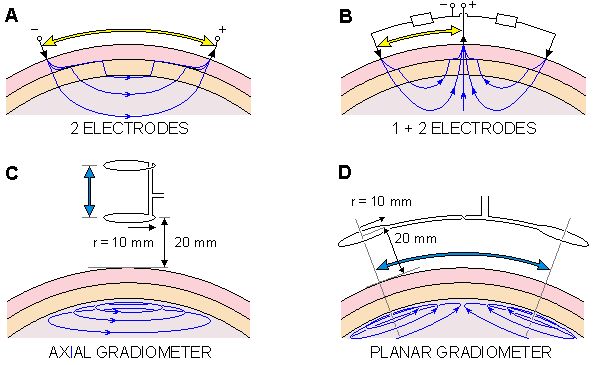D. Existing MEG Measurement Systems

In biomagnetic measurements two kind of detectors are used. These are axial and planar gradiometers.
Another important MEG instrument design is the micro-SQUID  in which the detector coil is situated in the vacuum space allowing measurements at very small measurement distances, being down to 1.5 mm from the scalp. The coil radius is approximately 1 to 2 mm. Such a device has only a few channels and it can be used only in a limited region of the head at a time.

In our calculations the axial gradiometer coil radius was 10 mm. The distance of the detector coil from the scalp was 20 mm being thus 32 mm from the surface of the brain. These dimensions are typical of whole-head multichannel MEG devices. The HSV was calculated as a function of the baseline (coil separation in the radial direction), Fig. 1(c). In MEG measurement devices the baselines usually vary between 30 and 100 mm. As discussed above, multichannel MEG devices built from axial gradiometers actually operate as planar gradiometers. Therefore, these calculations have more theoretical than practical value.
In the planar gradiometer calculations the coil radius was either 10 mm or 1 mm. For the 10 mm coil the measurement distance from the scalp was 20 mm, representing the whole-head MEG devices. The 1 mm coil radius represents the micro-SQUID device. For the measurement distance, values between 20 mm and 0 mm were used. The 0 mm distance is a theoretical minimum which is impossible to achieve with a superconducting device. Decreasing the coil radius below 1 mm would not significantly change the shape of the lead field at the measurement distances used . The HSV was again calculated as a function of the baseline, Fig. 1(d). The coils were coaxial with the spherical head model and the baseline could be expressed as an angle between the coils or distance along the scalp. The equations for calculating the magnetic lead fields are found from , , .
IV. RESULTS

A. The Forms of the Lead Fields of Electric and Magnetic Leads

The lead fields for two-electrode leads with 180°, 60° and 20° electrode separations are shown in Fig. 2(a), (b) and (c). The lead fields are displayed with lead field current flow lines (thin solid lines).² The illustration also includes the isosensitivity lines (dashed lines). The HSV's are indicated with shading. The insulating effect of the skull causes the sensitivity to distribute quite homogeneously within the brain region. At large electrode separations the sensitivity is directed mainly radially and at small electrode distances mainly tangentially to the head.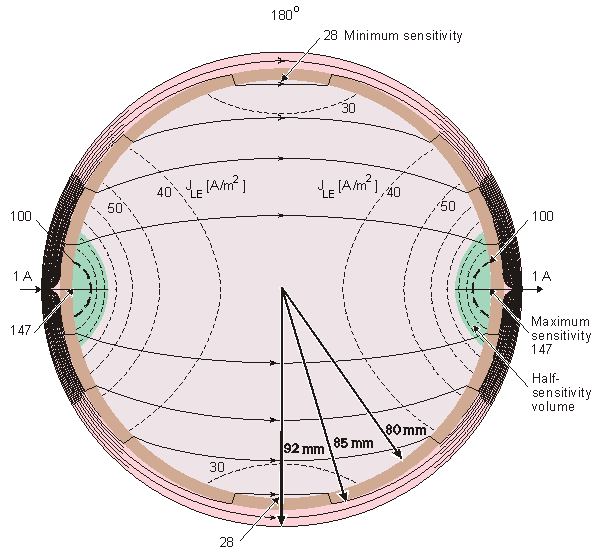Fig. 2. The EEG lead fields for two-electrode leads with (a) 180°, (b) 60° and (c) 20° electrode separations. The thin solid lines represent the lead field current flow lines. The dashed lines represent the isosensitivity surfaces. The HSV's are shown with shading.

² Note that in addition to representing the path of certain electrons, the lead field current lines represent the current density. Thus, the space between two lines represents a certain amount of current. Because the volume conductor thickness is larger in the center, the current density decreases more than it would decrease only on the basis of its width. Therefore, some of the lines have to end in the volume conductor region. If the thickness of the volume conductor were constant, all current lines would be continuous.
The lead field for the three-electrode lead is presented in Fig. 3. The sensitivity is mainly radial underneath the central electrode in the region of the HSV. This holds true at all electrode separations although the lead field current flow lines spread more when the electrode separation is small. When the separation of the distal electrodes in the three-electrode lead reaches 360°, it is identical to the two-electrode lead with 180° electrode separation.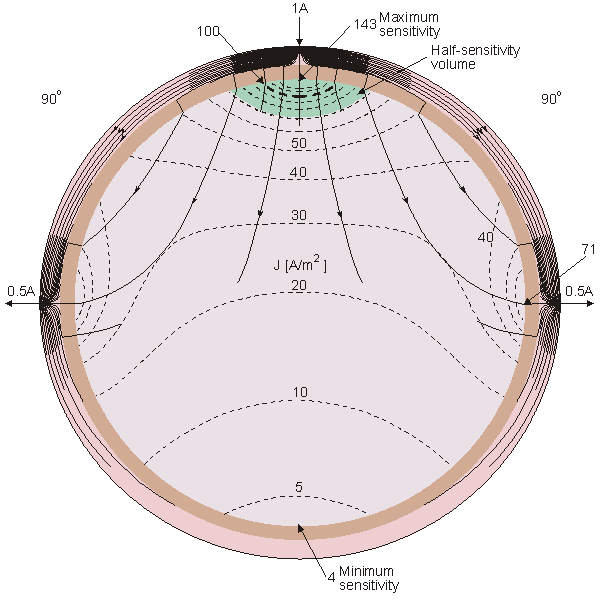Fig. 4 illustrates the lead field for an axial gradiometer. It has a tangential sensitivity distribution throughout the brain region. The sensitivity is zero at the symmetry axis. This is called zero sensitivity line  and it is represented by a thick dashed line. The sensitivity increases as a function of the radial distance from the symmetry axis.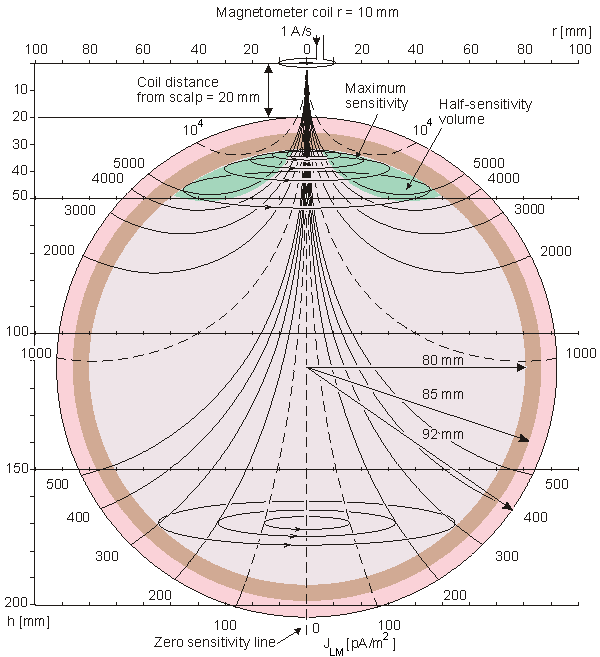Fig. 4. The lead field for an axial magnetometer with 10 mm coil radius and 20 mm measurement distance from the scalp. The lead field, shown with thin solid lines, is everywhere tangential to the head model and circulates around the symmetry axis which is drawn as a thick dashed line. The sensitivity is zero on the symmetry axis and it is therefore called the zero sensitivity line. The thin dashed lines represent the isosensitivity surfaces.

Fig. 5(a) and (b) illustrate the form of the lead field for a planar gradiometer in two orthogonal planes. Because all magnetic leads in spherical volume conductors are tangentially oriented, the planar gradiometer also has a sensitivity distribution tangential to the spherical head model surface. Because the coil configuration is quadrupolar when the baseline is small, the sensitivity has its maximum value under the common center of the two coils. The sensitivity is linear in the region of the HSV. In Fig. 6 this is illustrated with lead sensitivity vectors calculated in an infinite homogeneous volume conductor at two planes , .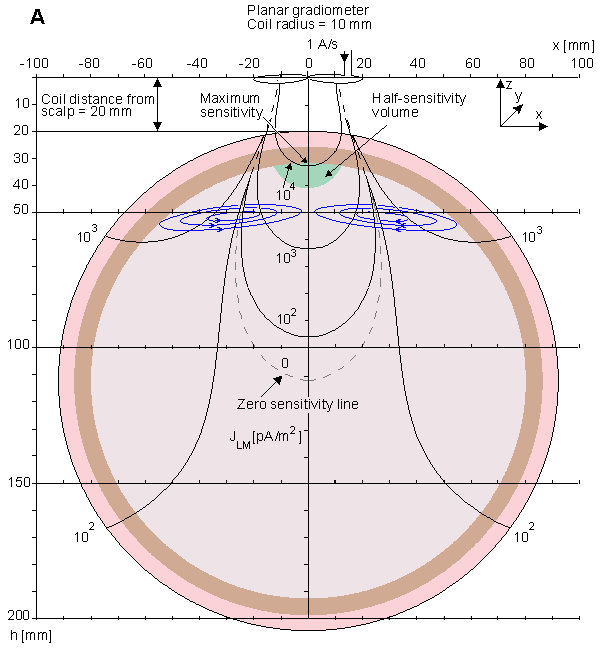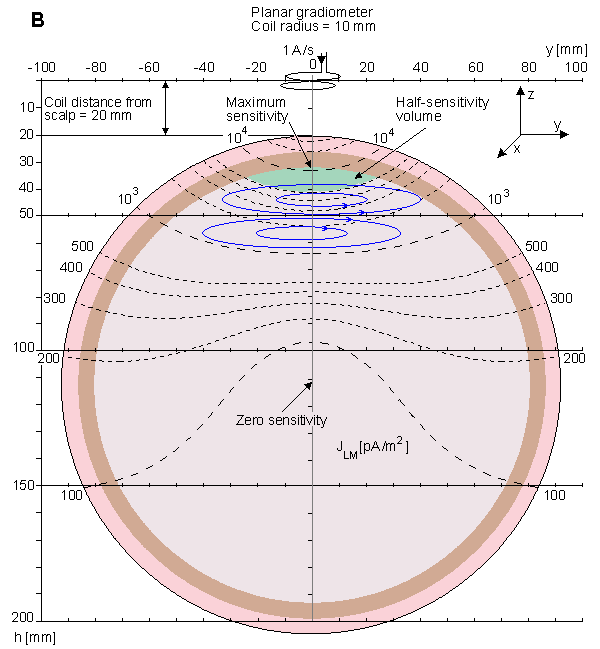Fig. 5.The lead field for a planar gradiometer with 10 mm coil radii and 20 mm baseline shown in two planes: (a) in the plane of the coil axes and (b) in the plane normal to that. The approximated lead field is shown with thin solid lines. It is everywhere tangential to the head model and has a quadrupolar form. The thin dashed lines represent the isosensitivity surfaces. The thick dashed line is the zero sensitivity line.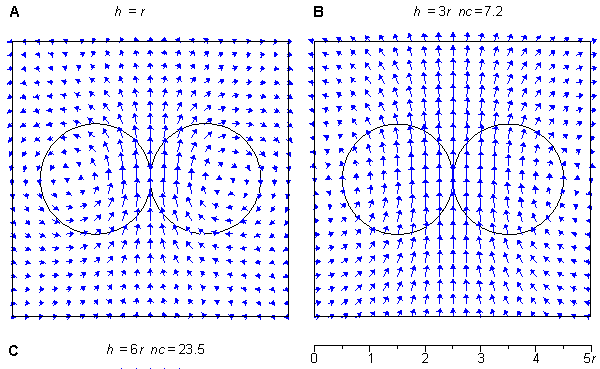Fig. 6. The lead field for a planar gradiometer in a plane at a distance of (a) one coil radius and (b) three coil radii from the coil plane. In (b) the lead field current density vectors are multiplied by factor of 7.2, compared to (a) to make them observable. This result is calculated for an infinite homogeneous volume conductor.

With electric leads it is possible to detect both radially and tangentially oriented sources. Because the lead field flow lines of all magnetic leads are always tangential to the spherical volume conductor, the magnetic leads detect only sources oriented tangentially to the head. Thus, from the three orthogonal components of every source, all three can be detected with electric leads but only the two tangential ones can be detected with magnetic leads; see Fig. 7.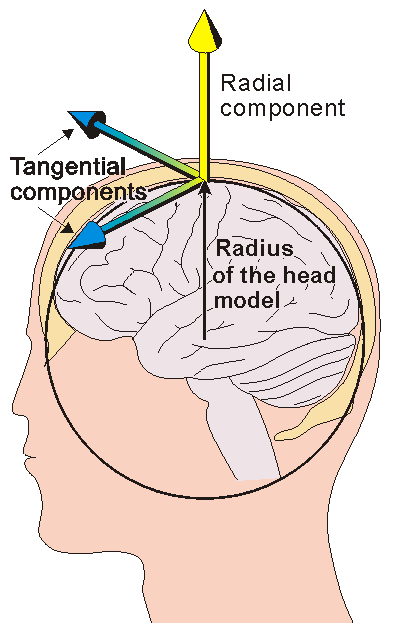Fig. 7. The EEG is sensitive to all the three components of the electric activity of the brain. The MEG is sensitive only to the two tangential components.

B. Half-Sensitivity Volumes of Electric and Magnetic Leads

1) Display of the Results: The HSV's for electric and magnetic leads are displayed as a function of detector separation in Fig. 8. The detector separation is given both in degrees and in circumferential distance on the scalp. For axial gradiometers the baseline is given on the same separation scale, though the distance between the coils is not measured along the scalp but in the radial direction. The HSV's are given in cm3 of brain region volume. Fig. 8(a) illustrates the results for baselines 0° - 180°. The most interesting region, that where the baselines are 0° - 20°, is illustrated in Fig. 8(b).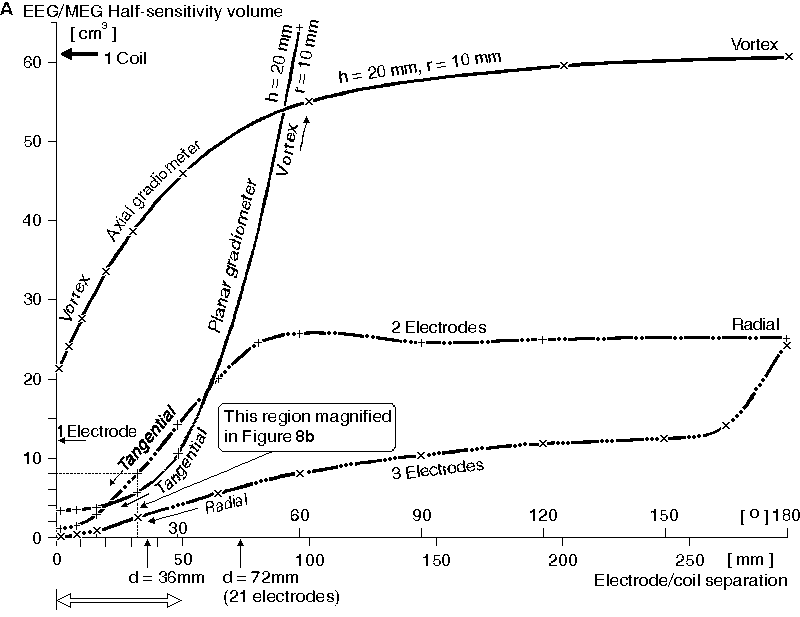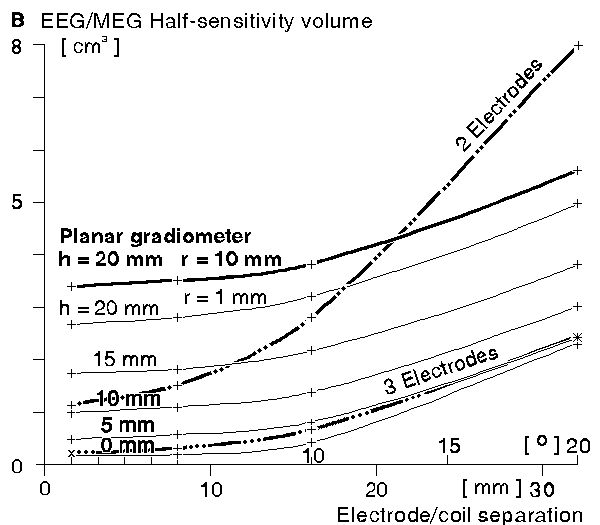2) Half-Sensitivity Volumes in the Standard Head Model: With the two-electrode lead at 1° separation an HSV of 1.2 cm³ is obtained. Then it increases as a function of separation having its maximum at about 60° separation. From there it decreases slightly, being 25 cm³ at 180° separation; see Fig. 8 and Table I.

TABLE I.
HSV OF TWO- AND THREE-ELECTRODE EEG LEADS AND AXIAL AND
PLANAR GRADIOMETER MEG LEADS WITH h = 20 mm, r = 10 mm GIVEN IN [cm³].

Separation EEG MEG
2-electr. 3-electr. Axial Planar
20° 32 mm 8.0 2.4 39 5.6
10° 16 mm 2.8 0.67 31 3.8
5° 8.0 mm 1.5 0.3 26 3.5
1° 1.6 mm 1.2 0.21 22 3.4

At small baselines the HSV of the three-electrode lead is 0.2 cm³, i.e., 1/6 of the two-electrode lead. The HSV increases monotonically as a function of electrode separation. When the electrode separation reaches 180° the HSV is equal to that of the two-electrode arrangement.
The HSV of an axial gradiometer with h = 20 mm and r = 10 mm has its minimum at minimum coil separation and increases as a function of coil separation, approaching that of a single coil magnetometer. At 1.6 mm coil separation, the HSV of the axial gradiometer is 22 cm³, see Table I. At 300 mm coil separation it is 61 cm³.
At small coil separations the HSV of a planar gradiometer with h = 20 mm and r = 10 mm is much smaller than that of the axial gradiometer, being 3.4 cm³ at 1° coil separation, as shown in Table I. At larger than about 20° separation the planar gradiometer HSV starts to increase rapidly and at very large separation it is about twice that of the axial gradiometer.
Decreasing the planar gradiometer coil radius to r = 1 mm decreases the HSV at 1° coil separation approximately 20% to 2.7 cm³, as illustrated in Fig. 8(b) and Table II. Decreasing the measurement distance to h = 15, 10, 5, and 0 mm decreases the HSV at 1° coil separation to 1.7, 0.97, 0.48 and 0.16 cm³, respectively. As discussed earlier, the measurement distance h = 0 mm cannot be achieved with superconducting technology, but it represents the theoretical minimum measurement distance.

TABLE II.
HSV OF PLANAR GRADIOMETERS WITH h = 0 ... 20 mm, r = 1 mm GIVEN IN [cm³].

20 mm 15 mm 10 mm 5 mm 0 mm
20° 5.0 3.8 3.0 2.4 2.3
10° 3.2 2.2 1.4 0.8 0.41
5° 2.8 1.8 1.1 0.55 0.19
1° 2.7 1.7 0.97 0.48 0.16

3) Effect of Skull Conductivity on the Half-sensitivity Volumes:Some uncertainty exists in the literature concerning the conductivity of the skull. We investigated the effect of the skull conductivity on the HSV's of the electric leads by decreasing it by 25% from 1/80 to 1/100 times the conductivities of the brain and the scalp. These results are illustrated in Fig. 9 and Table III. Below 5° electrode separation, its effect on the HSV was marginal. At 20° electrode separation the HSV was increased only about 10%.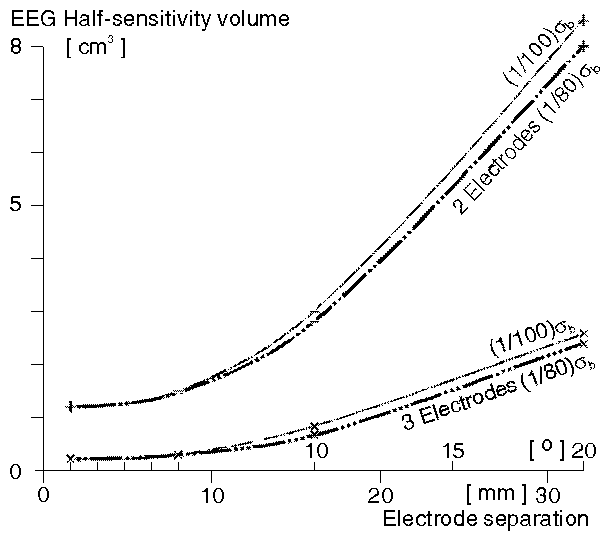Fig. 9. The effect of skull conductivity on the HSV's of the two- and three-electrode EEG leads. The results are calculated with skull conductivities of (1/80) sigma b and (1/80) sigma b where sigma b is the conductivity of brain (and scalp).

TABLE III.
EFFECT OF SKULL CONDUCTIVITY ON THE HSV OF TWO- AND THREE-ELECTRODE EEG LEADS.
HSV'S ARE GIVEN IN [cm³] WITH (1/80) sigma b AND (1/100) sigma b SKULL CONDUCTIVITIES.

Separation 2-electrode EEG 3-electrode EEG
(1/80)sigma b (1/100)sigma b (1/80)sigma b (1/100)sigma b
20° 8.0 8.5 2.4 2.6
10° 2.8 3.0 0.67 0.89
5° 1.5 1.5 0.3 0.3
1° 1.2 1.2 0.21 0.22

In a spherical model the skull conductivity does not have any effect on the lead fields of the magnetic leads.

4) Effect of the distance between the cortex and the skull on the half-sensitivity volumes: In the real head the cortex does not touch the skull but there is a space filled by cerebrospinal fluid. We investigated the effect of the distance between the cortex and the skull (i.e., the cerebrospinal fluid) by using values 0 mm, 2 mm and 4 mm to represent this distance. We used for the cerebrospinal fluid the conductivity of three times that of the brain being sigma c = 1.35 (Ohm·m)-1. The corresponding HSV's for the electric and magnetic leads are shown in Fig. 10 and Table IV. The effect is quite remarkable in the electric leads. At 1° separation the 2 and 4 mm distances magnified the HSV by factors of 2.7 and 4.4 for the two-electrode lead and by factors 2.4 and 3.5 for the three-electrode lead, respectively. When the separation increases, the relative increase of the HSV decreases. In Table IV we have also given the HSV's calculated with the same CSF conductivity as that of the brain to give the reader a possibility to estimate the effect of the CSF conductivity.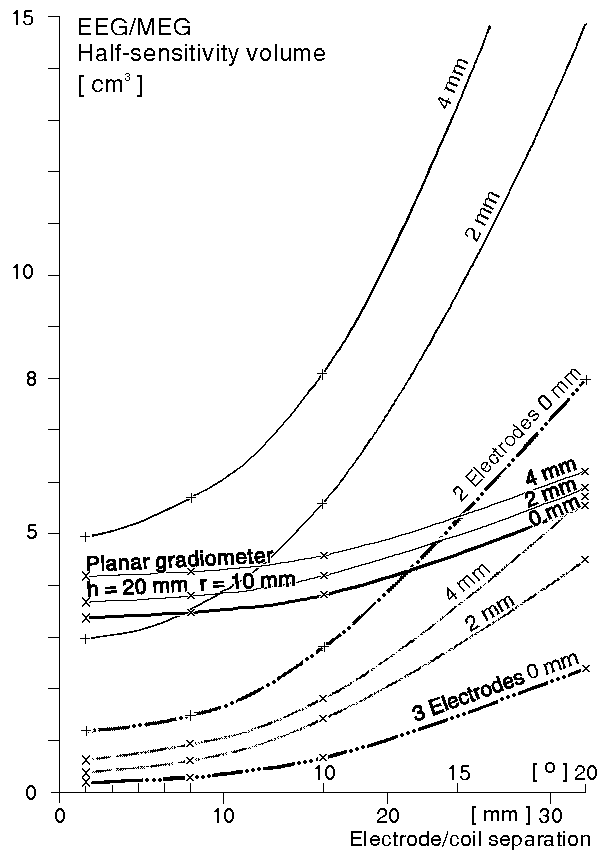Fig. 10. The effect of the distance of the cortex from the skull on the HSV's of electric and magnetic leads. The HSV's of two- and three-electrode electric leads and of planar and axial gradiometers plotted as a function of electrode distance/gradiometer coil separation. The results are calculated for 0 mm, 2 mm and 4 mm distances between skull and cortex.

TABLE IV.
EFFECT OF THE DISTANCE BETWEEN THE CORTEX AND THE SKULL TO THE HSV OF
HSV'S ARE GIVEN IN [cm³] FOR 0-mm, 2-mm AND 4-mm DISTANCES
BETWEEN THE CORTEX AND THE SKULL.
CONDUCTIVITY OF THE CSF IS sigma c = 1.35 (Ohm·m)-1 BEING THREE TIMES THAT OF THE BRAIN AND SCALP. RESULTS ARE ALSO CALCULATED FOR sigma c = sigma t = sigma b.

Separation 2-electrode EEG 3-electrode EEG

sigma c = 1.35 sigma b = 0.45
sigma c = 1.35 sigma b = 0.45
0 mm 2 mm 4 mm 2 mm 4 mm 0 mm 2 mm 4 mm 2 mm 4 mm
20° 8.0 15.6 20.6 11 14.3 2.4 4.7 5.9 3.6 4.6
10° 2.8 5.9 8.6 4.1 5.7 0.67 1.5 2.0 1.2 1.5
5° 1.5 3.8 6.1 2.4 3.9 0.3 0.72 0.98 0.51 0.77
1° 1.2 3.2 5.3 2.0 3.4 0.21 0.5 0.74 0.3 0.57

As shown in Fig. 10 and Table V, in the magnetic leads the effect is smaller. (Because the vertical scale in Fig. 10 is the same as in Figs. 9 and 8, the HSV's of the axial gradiometer at 2 and 4 mm distance between the cortex and the skull did not fit to the figure and they can be found only from Table V.) At 1° separation the 2 and 4 mm distances magnified the HSV by factors of 1.07 and 1.2 for the planar gradiometer lead and by factors 1.2 and 1.3 for the axial gradiometer lead, respectively. When the separation increases the relative increase of the HSV slightly decreases. (The CSF conductance does not have any effect to the HSV in a magnetic lead.)

TABLE V
EFFECT OF THE DISTANCE BETWEEN THE CORTEX AND THE SKULL TO THE HSV OF AXIAL AND PLANAR GRADIOMETERS WITH h = 20 mm, r = 10 mm. HSV's ARE GIVEN IN [cm³] for 0, 2, and 4 mm DISTANCES BETWEEN THE CORTEX AND THE SKULL.

0 mm 2 mm 4 mm 0 mm 2 mm 4 mm
20° 32 mm 39 44 48 5.6 5.9 6.2
10° 16 mm 31 34 38 3.8 4.2 4.6
5° 8.0 mm 26 30 33 3.5 3.8 4.3
1° 1.6 mm 21 25 28 3.4 3.6 4.2

C. Maximum Sensitivities of Electric and Magnetic Leads

1) Maximum Sensitivities in the Standard Head Model: When the electrode separation decreases, the maximum sensitivity of the electric leads decreases from amaximum of JLE = 147 A/m² at 180° separation to 11 A/m² and 1.5 A/m² for the two- and three-electrode leads at 1° separation, respectively. It reaches the -3 dB values at about 18.5° and 25° separations, respectively, Fig. 11(a) and Table VI.Fig. 11. The maximum sensitivity of (a) the two- and three-electrode EEG leads and b) the MEG leads of axial and planar gradiometers with coil radii of 10 mm plotted as a function of electrode distance/gradiometer separation.

TABLE VI
MAXIMUM SENSITIVITIES OF TWO- AND THREE-ELECTRODE EEG AND OF AXIAL AND PLANAR GRADIOMETERS WITH h = 20 mm, r = 10 mm. SENSITIVITIES FOR THE ELECTRIC LEADS ARE GIVEN IN [A/m²] WHILE THESE FOR THE MAGNETIC LEADS ARE GIVEN IN [nA/m²].

Separation 2-electrode

[A/m²]
3-electrode

[A/m²]
Axial
[nA/m²]
Planar
[nA/m²]
180° 289 mm 150 150 9.2 9.6
90° 145 mm 140 140 9.1 11
30° 48 mm 120 110 8.2 19
20° 32 mm 108 97 7.5 17
10° 16 mm 82 62 5.5 11
5° 8.0 mm 51 28 3.6 5.7
1° 1.6 mm 11 1.5 0.89 1.2

The sensitivity of the magnetic leads is illustrated in Fig. 11(b) and Table VI. The maximum sensitivity of axial gradiometers at large coil separation approaches the value obtained for a single coil magnetometer and is equal to 9.3 µA/m². It reaches the -3 dB value at a coil separation of approximately 23 mm. The planar gradiometer has its maximum sensitivity at about 30° coil separation and reaches the -3 dB value at about 14° separation.

2) Effect of Skull Conductivity on the Maximum Sensitivities: Fig. 12 and Table VII illustrate the effect of the skull conductivity on the maximum sensitivity of the electric leads when decreasing its value by 25%. At 1° baseline the maximum sensitivity of two- and three-electrode EEG's decreases some 15%, at 20° baseline the decrease is about 18%.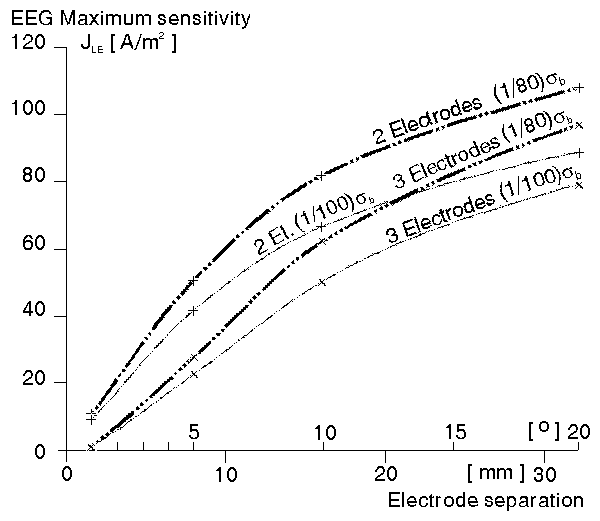Fig. 12. The effect of the skull conductivity on the maximum sensitivity of the two- and three-electrode EEG-leads. The results are calculated with skull conductivities of (1/80) sigma b and (1/100) sigma b where sigma b is the conductivity of brain (and scalp).

TABLE VII.
EFFECT OF THE SKULL CONDUCTIVITY ON THE MAXIMUM SENSITIVITY OF TWO- AND THREE-ELECTRODE EEG LEADS GIVEN IN [A/m²] WITH (1/80) sigma b AND (1/100) sigma b SKULL CONDUCTIVITIES.

Separation 2-electrode EEG 3-electrode EEG
(1/80)sigma b (1/100)sigma b (1/80)sigma b (1/100)sigma b
20° 110 89 97 79
10° 82 67 63 50
5° 51 42 28 23
1° 11 9.2 1.5 1.3

In a spherical model the skull conductivity does not have any effect on the maximum sensitivity of the magnetic leads.

3) Effect of the Distance Between the Cortex and the Skull on the Maximum Sensitivities: At 1° separation, changing the distance between the cortex and the skull from 0 mm to 2 and 4 mm decreases the maximum sensitivity of two-electrode lead some 48% and 65% and three-electrode lead some 64% and 79%, respectively. At 20° separation the relative decrease is smaller, see Fig. 13(a) and Table VIII.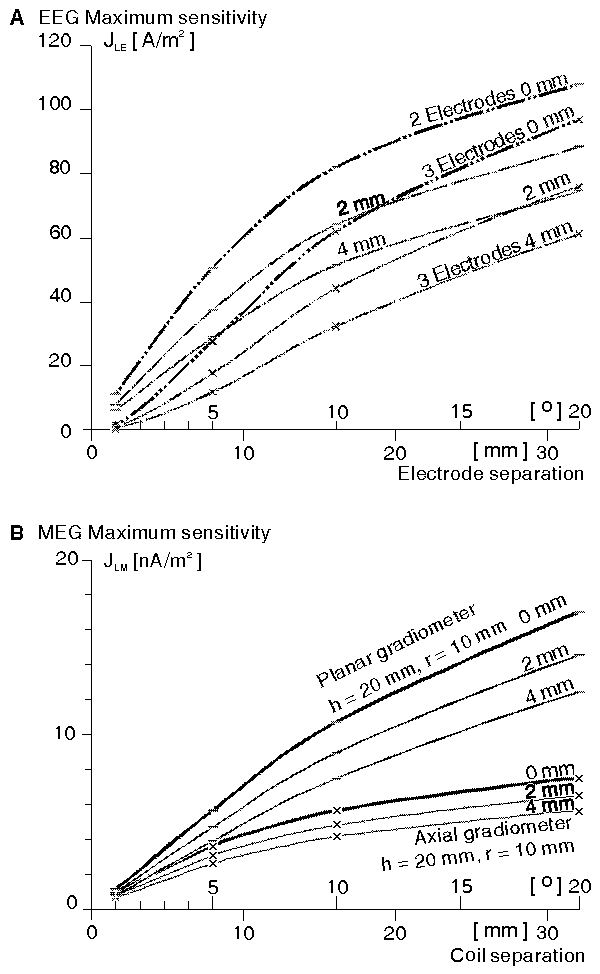Fig. 13. The effect of the distance between the cortex and the skull on the maximum sensitivity of electric and magnetic leads. (a) Maximum sensitivities of two- and three-electrode electric leads. (b) Maximum sensitivities of planar and axial gradiometers. The results are calculated for 0 mm, 2 mm and 4 mm distances between the cortex and the skull.

TABLE VIII
EFFECT OF THE DISTANCE BETWEEN THE CORTEX AND THE SKULL ON THE MAXIMUM SENSITIVITY OF TWO- AND THREE-ELECTRODE EEG LEADS GIVEN IN [A/m²] FOR DISTANCES OF 0 mm, 2 mm AND 4 mm BETWEEN THE CORTEX AND THE SKULL. THE CONDUCTIVITY OF THE CSF sigma c = 1.35 (Ohm·m)-1.

Separation 2-electrode EEG 3-electrode EEG
0 mm 2 mm 4 mm 0 mm 2 mm 4 mm
20° 32 mm 110 67 51 97 56 39
10° 16 mm 82 47 34 62 30 19
5° 8.0 mm 51 27 19 28 12 6.9
1° 1.6 mm 11 5.7 3.9 1.6 0.58 0.33

In the same situation the maximum sensitivity of axial gradiometer decreases some 16% and 29% and that of planar gradiometer some 20% and 33%, respectively. At 20° separation the relative decrease is a little smaller, as shown in Fig. 13(b) and Table IX.

TABLE IX
EFFECT OF THE DISTANCE BETWEEN THE CORTEX AND THE SKULL ON THE MAXIMUM SENSITIVITY OF AXIAL AND PLANAR GRADIOMETERS WITH h = 20 mm, r = 10 mm GIVEN IN [nA/m²] FOR DISTANCES 0 mm, 2 mm, AND 4 mm OF AXIAL AND PLANAR GRADIOMETERS WITH h = 20 mm, r = 10 mm GIVEN IN [nA/m²] FOR DISTANCES 0 mm, 2mm, AND 4 mm .

0 mm 2 mm 4 mm 0 mm 2 mm 4 mm
20° 32 mm 7.5 6.5 5.6 17 15 12
10° 16 mm 6.6 4.8 4.2 11 8.9 7.5
5° 8.0 mm 3.6 3.1 2.6 5.7 4.7 3.9
1° 1.6 mm 0.94 0.79 0.67 1.2 0.96 0.8

V. DISCUSSION

A. Forms of the Lead Fields

The radially oriented lead field of a single electrode electric lead cannot be synthesized with any magnetic leads. Similarly, the vortex-form lead field of an axial gradiometer cannot be synthesized with any electric leads. Thus, these leads give most complementary information about the electric sources of the brain. However, the axial gradiometer has very large HSV, as seen from Fig. 8(a), and its signal is thus a spatial average from a large region of the brain. Therefore it has very low clinical value.
With short electrode and coil separations, respectively, the lead fields of the two-electrode lead and the planar gradiometer closely resemble each other. Thus, the information from these leads is most redundant.

B. Half Sensitivity Volumes

Despite the diffusing effect of the skull the electric leads have smaller HSV's than the typical whole-head MEG systems. Even with the smallest possible coil separations, the planar gradiometer requires recording distances of 12 mm or less to reach the HSV of the two-electrode EEG lead. The three-electrode EEG-leads are superior, having HSV comparable to the theoretical 0 mm micro-SQUID planar gradiometer measurement distance.

C. Maximum Sensitivities

When the electrodes or coils are brought closer to each other the HSV becomes smaller. While this improves the form of the lead field, it also decreases the sensitivity of the lead. The important question is whether the signal amplitude is acceptable at those separations where the HSV is sufficiently small.
In electric leads the -3 dB signal amplitude is reached with larger separation than for magnetic leads. This result means that if identical electrode/coil separations are used in both electric and magnetic leads, attenuation from the maximum amplitude with electric signal is greater than with the magnetic signal. However, because the signal-to-noise ratio of the electric measurement is much greater than in the magnetic one, the electric measurement is much easier to perform also with shorter electrode separation.

D. Effect of the Parameters of the Head Model

A given percentage change in the conductivity of the skull causes a smaller percentage change in the maximum sensitivities and HSV's of the electric leads. On the magnetic leads it does not have any effect. Thus, changing the skull conductivity is not an important factor.
The distance between the cortex and the skull, i.e., the effect of the cerebrospinal fluid, has a large effect on the results. It makes the HSV's larger and the signal amplitudes smaller in both electric and magnetic leads. The effect on HSV is larger in electric leads than it is in magnetic leads.
VI. CONCLUSION

Our theoretical analysis shows the following.
1) With the EEG it is possible to record the electric activity of the brain from a more concentrated region than with the MEG
2) The sensitivity distributions of such MEG leads which have small HSV's (planar gradiometers) resemble very much those of dipolar EEG leads and therefore they record the electric activity of the brain in a very similar way
3) The effect of head-model parameters is either small or at least similar in both EEG and MEG leads.

Furthermore it is known that the EEG can record the three orthogonal components of the electric sources in the cortex but the MEG can record only the two tangential ones.
In biomagnetic research, recordings are not consistently compared with corresponding electric recordings. Therefore it is not usually shown that the results obtained with magnetic methods could have been obtained equally well or even more accurately with electric methods. To assess the clinical diagnostic value of the MEG and to be able to justify its much higher price compared with electric methods, MEG studies should be performed parallel with EEG studies.
APPENDIX

EQUATIONS FOR CALCULATING ELECTRIC LEAD FIELDS
AND HALF-SENSITIVITY VOLUMES
IN A FOUR CONCENTRIC-SPHERES MODEL

Assuming solution of the form V = f(r)g(q)h(f) the general solution can be shown to be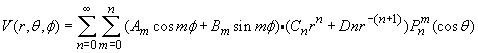(A.1)

where Pnm(cosf) is the associated Legendre polynomial of the first kind. The coefficients Am , Bm , Cn and Dn are determined from boundary conditions.

For any arbitrary placement of electrodes on the surface of the outer sphere, the coordinate system can always be oriented in such way that f will have only even symmetry about f = 0. Thus the coefficients of sinmf will be zero. Constant terms representing uniform fields will be neglected and term Dn r - (n+1) will be omitted in the innermost sphere (brain), since the negative powers of r would cause a singularity at the origin.
Boundary conditions: The normal component of the current density on the outermost surface must be zero everywhere except at the electrodes. In these equations the subscripts have the following meaning: t = scalp, s = skull, c = CSF, and b = brain.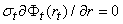(A.2)

Furthermore, the normal component of the current density and the potential must be equal everywhere on the corresponding surfaces of the inner spheres.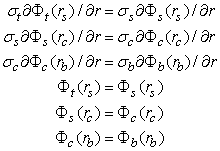(A.3)

The boundary conditions give seven equations with seven unknowns.
The solution for the potential in the brain can be shown to be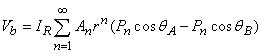(A.4)

where

IR = reciprocal current fed to the electrodes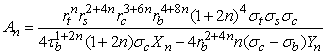in whichand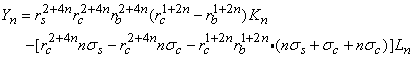where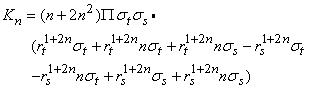andREFERENCES

 S. Abboud, Y. Eshel, S. Levy, M. Rosenfeld, "Numerical calculation of the potential distribution due to dipole sources in a spherical model of the head," Comput. Biomed. Res., vol. 27, pp. 441-455, 1994.

 M. Balish, S. Sato, P. Connaughton, C. Kufta, "Localization of implanted dipoles by magnetoencephalography," Neurology, vol. 41, pp. 1072-1076, 1991.

 Biomagnetic Technologies Inc., San Diego, California.

 D. Cohen, B.N. Cuffin, K. Yunokuchi, R. Maniewski, C. Purcell, G.R. Cosgrove, J. Ives, J.G. Kennedy, D.L. Schomer, "MEG versus EEG localization test using implanted sources in the human brain," Ann. Neurol., vol. 28, pp. 811-817, 1990.

 R.P. Crease, "Images of conflict: MEG vs. EEG," Science, vol. 253, (26) pp. 374-375, 1991.

 B.N. Cuffin, D. Cohen, K. Yunokuchi, R. Maniewski, C. Purcell, G.R. Cosgrove, J. Ives, J. Kennedy, D. Schomer, "Tests of EEG localization accuracy using implanted sources in the human brain," Ann. Neurol., vol. 29, pp. 132-138, 1991.

 Y. Eshel, S.L. Witman, M. Rosenfeld, S. Abboud, "Correlation between skull thickness asymmetry and scalp potential estimated by a numerical model of the head," IEEE Trans. Biomed. Eng., vol. 42, pp. 242-249, 1995.

 H.J. Eskola, J.A. Malmivuo, J.J. Nousiainen, J.O. Lekkala, "Corrected unipositional lead system for vector magnetocardiography," IEEE Trans. Biomed. Eng., vol. BME-34, pp. 81-90, 1987.

 H.L.F. Helmholtz, "Über einige Gesetze der Vertheilung elektrischer Ströme in körperlichen Leitern mit Anwendung auf die thierisch-elektrischen Versuche," Ann. Physik und Chemie, vol. 89, pp. 211-233, 354-377, 1853.

 J.A. Malmivuo, On the detection of the magnetic heart vector - An application of the reciprocity theorem. Helsinki Univ. Tech., Acta Polytechn. Scand., El. Eng. Series. Vol. 39., pp. 112, (Dr. tech. thesis), 1976.

 J.A. Malmivuo, "Distribution of MEG detector sensitivity: An application of reciprocity," Med. & Biol. Eng. & Comput., vol. 18, pp. 365-370, 1980.

 J. Malmivuo "Sensitivity distribution of MEG measurement and energy distribution in magnetic stimulation of the central nervous system", in Proc. of the 2nd Far Eastern Conf. Medical and Biological Engineering, August 15-18, Beijing, p. 370, 1993.

 J. Malmivuo, R. Plonsey, Bioelectromagnetism - Principles and applications of bioelectric and biomagnetic fields, pp. 480, Oxford University Press, New York, 1995.

 J.A. Malmivuo, J. Puikkonen, "Sensitivity distribution of multichannel MEG detectors", in Abstr. 6th Internat. Conf. Biomagnetism, Tokyo, 27-30 August, ed. Atsumi K, Kotani M, Ueno S, Katila T, Williamson SJ, pp. 112-3, Tokyo Denki University Press, Tokyo, 1987.

 J.A. Malmivuo, J. Puikkonen, "Qualitative properties of the sensitivity distribution of planar gradiometers," Tampere Univ. Techn., Inst. Biomed. Eng., Rep. vol. 2, pp. 35, 1988.

 J. Puikkonen, J.A. Malmivuo, "Theoretical investigation of the sensitivity distribution of point EEG-electrodes on the three concentric spheres model of a human head - An application of the reciprocity theorem," Tampere Univ. Techn., Inst. Biomed. Eng., Rep., vol. 1, pp. 71, 1987.

 S. Rush, D.A. Driscoll, "EEG-electrode sensitivity - An application of reciprocity," IEEE Trans. Biomed. Eng., vol. BME-16, pp. 15-22, 1969.

 W.R. Smythe, Static and dynamic electricity, 3rd ed., pp. 623, McGraw-Hill, New York, N.Y., 1968.

 C.J. Stok, "The influence of model parameters on EEG/MEG single dipole source estimation," IEEE Trans. Biomed. Eng., vol. BME-34, pp. 289-296, 1987.

 V. Suihko, H. Eskola, J.A. Malmivuo, "Effect of electrode size in electroencephalography and electrical transcranial stimulation," in Proc. 1st Int. Conf. on Medical Physics & Biomedical Engineering, Nicosia, Cyprus, pp. 306-310, 1994.

 V. Suihko, J. Malmivuo, "Sensitivity distributions of EEG and MEG measurements," in EEG and MEG Signal Analysis and Interpretation, Proc. 2nd Ragnar Granit Symp., ed. J. Hyttinen, J. Malmivuo, pp. 11-20, Tampere University of Technology, Ragnar Granit Institute, Tampere, 1993.

 V. Suihko, J.A. Malmivuo, H. Eskola, "Distribution of sensitivity of electric leads in an inhomogeneous spherical head model," Tampere Univ. Techn., Ragnar Granit Inst., Rep., vol. 7, 1993.

 S. Tan, B.J. Roth, J.P. Wikswo, "The magnetic field of cortical current sources: the application of spatial filtering model to the forward and inverse problems," Electroenceph. Clin. Neurophysiol., vol. 76, pp. 73-85, 1990.

 J.P. Wikswo, A. Gevins, S.J. Williamson, "The future of the EEG and MEG," Electroenceph. Clin. Neurophysiol., vol. 87, pp. 1-9, 1993.

Acknowledgement. This work has been supported by the Academy of Finland and the Ragnar Granit Foundation.Jaakko Malmivuo (S'71-M'92-SM'95) received the M.Sc. and Ph.D. degrees from Helsinki University of Technology, Espoo, Finland, in 1971 and 1976, respectively. From 1974 to 1976 he served as researcher at Stanford University. In 1976 he was appointed as Associate Professor and in 1986 Professor of Bioelectromagnetism at Tampere University of Technology (TUT), Tampere, Finland. Since 1992 he has been Director of the Ragnar Granit Institute at TUT. He has served as Visiting Professor at Technical University of Berlin (West) (1988), Dalhousie University, Halifax, Canada (1989), and Sophia University, Tokyo, (1993). He has over 250 scientific publications and he has co-written with R. Plonsey, Bioelectromagnetism, (New York; Oxford Univ. Press, 1995). Dr. Malmivuo was President of the Finnish Society for Medical Physics and Medical Engineering in 1987-1990. He is founder Member and President of the International Society for Bioelectromagnetism.Veikko E. Suihko received the M.Sc. degree in electrical engineering and the Lic.Tech. degree in biomedical engineering from Tampere University of Technology, Tampere, Finland, in 1991 and 1994, respectively. From 1991 to 1996, he was researcher at the Ragnar Granit Institute at Tampere University of Technology. He is currently Associate Physicist at Tampere University Hospital. His research interests include modeling of bioelectric phenomena with applications to noninvasive electric stimulation of central nervous system and measurement of electric activity of the brain.Hannu Eskola was born in Honkilahti, Finland, on November 13, 1954. He received the M.Sc. and Ph.D. degrees in biomedical engineering from Tampere University of Technology, Tampere, Finland, in 1979 and 1983, respectively. Since 1980 he has been Hospital Physicist in various departments in University Hospitals of Tampere and Kuopio. In 1994 he joined the Ragnar Granit Institute at Tampere University of Technology and became Associate Professor in Medical Electronics. His main research interests are in neurophysiology, especially in modelling of electric fields in the brain.